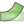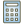# Adding and visualizing point data

Hi all,

In advance I’d like to apologize for my lack of in-depth knowledge regarding Paraview.

GOAL
I’m trying to visualize the motion of a mesh, consisting of two separate data sets: (1) general displacement and (2) deformation (both xmf / hdf5 formatted). These data sets have the same topology, but different spatial values.

The closest I got to a solution was to use the Python Calculator filter as described in . Using the spreadsheet view, I can see the point data is added correctly, but I don’t know how to use the result to create a new mesh object.

I can assign the result to cells, but that would means they show up as a color, rather than actual displacement.

So it sounds like you have used the `Python Calculator` to create a point field that describes how each point moves. I assume that means you have an x,y,z vector that describes how each point moves. You say you can see that in the spreadsheet. You should also be able to color by that vector.

I’ve already guessed quite a bit on what you have so far. Assuming I’ve guessed correctly, I’m still not sure if these point vectors represent a displacement (i.e. how far to move each point) or a point coordinate (an absolute position on where to put each point).

If what you have are displacements, you can use the `Warp By Vector`filter. This filter will add your displacements to each point coordinate to put them in the position you want.

If what you have are absolute positions, you can use the `Calculator`filter. Just turn on the `Coordinate Results` checkbox and set the expression to be the vector field you created.

Data set 1 = location + displacement(t) (these are absolute values)
Data set 2 = deformation(t) (these are relative)

I import them by opening the .xmf part of the data sets. They show up in the pipeline and in the renderview as well. I indeed tried to take data set 1 and warp it by data set 2. But Warp by Vector option is deactivated.

Could it be that the warp by vector option is only allowed on non-temporal data sets? Meaning: we’d have to split up data set 1 into location and displacement and then apply warp by vector on the location part twice?

Thanks Kenneth, hopefully the problem is a bit clearer now.

It’s always helpful if you can post some example data if you can.

`Warp By Vector` should work fine with temporal data. (It just gets reapplied every time you go to a new timestep.) It does, however, require a data set with a point field of 3-component vectors (for the displacements). (It also has some limits on topology type.)

What it cannot do is pull in two data sets at a time. If you want to displace data set 1 by the displacements on data set 2, you first need to combine them. You should be able to do that with the `Append Attributes` filter if the two datasets have the same topology.

You’re right. I solved the problem by defining the displacement as an attribute rather than embedding it in the geometry. Using the xdmf formatting in , Paraview will recognize it as a point array which enables the Warp By Vector option.

Thanks! Next time I’ll be sure to add some example data.

 < Attribute Name=“displacement” Center=“Node” >